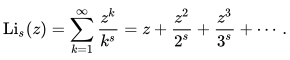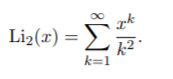# Polylogarithm Function, Dilogarithm

## Polylogarithm Function Definition

The polylogarithm function is a special function defined as:It is also called the Jonquiere function, and is defined over the complex plane and the open unit disk.

Polylogarithm functions are important in the computation of quantum electrodynamic corrections to the electrons gyromagnetic ratio, and because of that, two special cases have their own special name.
If n = 2, the polylogarithm is known as the dilogarithm; if n = 3, the trilogarithm.

## Properties of the Polylogarithm

If s is a nonpositive integer, the polylogarithm will be a rational function. For a fixed complex s and |z| <1, the polylogarithm is an analytic function, if |z| = 1 and s is a real number greater than 0, the series will converge (settle on a certain number).

## Alternative Names and Notations for the Polylogarithm Function

The polylogarithm function is most commonly abbreviated as Lis(z). Although the polylogarithm has a long history and was discussed in Euler, the notation is comparatively new and was introduced by mathematician Lewin, in 1981.

The dilogarithm, with s = 2, has also been abbreviated as s2(z) (by Kölbig et al in 1970) and L2(z) (by Maximon in 2003). In 1979, ‘t Hooft and Veltman called it the Spence Function and abbreviated it as Sp(z).

The general function was also referred to as φ(z, s) in 1945 (by Truesdall). It is sometimes called Jonquière’s function, for mathematician Alfred Jonquière who discussed it in 1889.

If z = 1, the polylogarithm has another special name: the Riemann zeta function: ζ (s) = Lis(1).

## Dilogarithm Functions

The dilogarithm function (sometimes called Euler’s dilogarithm function) is a special case of the polylogarithm that can be traced back to the works of Leonhard Euler. The function re-emerged in the latter half of the 20th century and is now popular in fields as diverse as algebraic topology, hyperbolic geometry, and in mathematical physics (for example, in conformal field theory and scissors congruence).

It’s one of the simplest non-elementary functions, but also “…one of the strangest” (Zagier, 2007).

## Dilogarithm Function Definition

The classical (Euler) dilogarithm function Li2 is defined for -1 ≤ x ≤ 1 by the power series:The radius of convergence for this series is 1; the definition is also valid in the unit disc of the complex plane (Distler, 2010).

You may also see it written as the following integral, valid for x ≤ 1 (Bressoud, 2006):Or, as an alternate (used in MATLAB):## Other Forms

A variant, the Bloch–Wigner dilogarithm is defined as follows:
D(z):= Im (Li2(z)) + arg(1 − z)log|z|
This is real-analytic in the complex plane from {0, 1} but vanishes on the real plane (i.e. for real numbers).

Rogers version is similar to the Bloch–Wigner, except that it is defined on ℙ1(ℝ.), where ℙ1 is the one-dimensional real projective space. Others include the “enhanced” (a combination of Rogers and Bloch-Wigner), double (two-argument), Faddeev & Kashaev’s quantum, p-adic, finite and modulo p. Describing all of these (somewhat obscure) functions is beyond the scope of this article, but you can find an excellent overview in Cartier et’ al’s book Frontiers in Number Theory, Physics, and Geometry II.

## References

Abramowitz, M. and Stegun, I. A. (Eds.). “Dilogarithm.” §27.7 in Handbook of Mathematical Functions with Formulas, Graphs, and Mathematical Tables, 9th printing. New York: Dover, pp. 1004-1005, 1972.
Aczel, J. (Ed.). Functional Equations: History, Applications and Theory (Mathematics and Its Applications). Springer, 1984.
Bressoud, D. A Radical Approach to Real Analysis 2nd edition. 2006.
[DLMF] NIST Digital Library of Mathematical Functions. http://dlmf.nist.gov/, Release 1.0.24 of 2019-09-15. F. W. J. Olver, A. B. Olde Daalhuis,
Distler, J. (2010). Geometry and String Theory: The Dilogarithm Function. Retrieved May 21, 2020 from: https://golem.ph.utexas.edu/wiki/gst/show/The+Dilogarithm+Function
Lewin, L. Dilogarithms and Associated Functions. London: Macdonald, 1958.
Lozier, D. et al., eds. Polylogarithms. Retrieved from https://dlmf.nist.gov/25.12 on November 19, 2019.
Zagier, D. (2007). The Dilogarithm Function. Retrieved May 21, 2020 from: https://people.mpim-bonn.mpg.de/zagier/files/doi/10.1007/978-3-540-30308-4_1/fulltext.pdf

CITE THIS AS:
Stephanie Glen. "Polylogarithm Function, Dilogarithm" From StatisticsHowTo.com: Elementary Statistics for the rest of us! https://www.statisticshowto.com/polylogarithm-function/